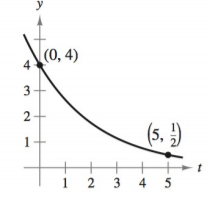Chapter 4.6, Problem 3E### Calculus: An Applied Approach (Min...

10th Edition
Ron Larson
ISBN: 9781305860919

#### Solutions

Chapter
Section### Calculus: An Applied Approach (Min...

10th Edition
Ron Larson
ISBN: 9781305860919
Textbook Problem
5 views

# Modeling Exponential Growth and Decay In Exercises 1-6, find the exponential function y = C e k t that passes through the two given points. y = C e k tTo determine

To calculate: The exponential function y=Cekt for the following graph:Explanation

Given Information:

The provided graph is:

Calculation:

Consider the following graph:

Observe that the exponential function curve passes through the points (0,4) and (5,12).

Hence the points (0,4) and (5,12) satisfy the equation of exponential function y=Cekt.

Substitute t=0,y=4 in the equation y=Cekt.

4=Cek(0)4=Ce04=C1C=4

Hence, the value of C is 4.

Substitute t=5,y=12 in the equation y=4ekt.

12=4ek(5)12=4e5k

Solve the equation 12=4e5k for k

### Still sussing out bartleby?

Check out a sample textbook solution.

See a sample solution

#### The Solution to Your Study Problems

Bartleby provides explanations to thousands of textbook problems written by our experts, many with advanced degrees!

Get Started

#### Solve the equations in Exercises 126. 1x211+1=0

Finite Mathematics and Applied Calculus (MindTap Course List)

#### In Exercises 69-74, rationalize the numerator. 71. 2yx

Applied Calculus for the Managerial, Life, and Social Sciences: A Brief Approach

#### Sketch the graph of the equation. 20. |y| = 1

Single Variable Calculus: Early Transcendentals

#### The length of the are r = e for 0 is given by: a) 0ed b) 02e2d c) 02ed d) 02ed

Study Guide for Stewart's Single Variable Calculus: Early Transcendentals, 8th